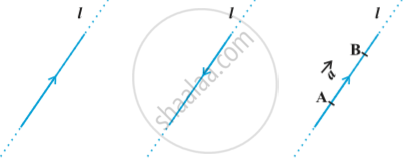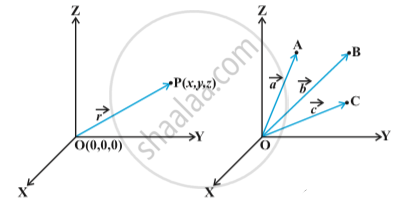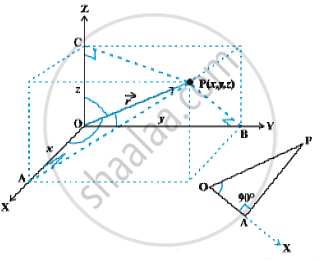# Basic Concepts of Vector Algebra

#### description

• Position Vector
• Direction Cosines and Direction Ratios of a Vector

#### definition

A quantity that has magnitude as well as direction is called a vector.
A directed line segment is a vector in above  fig., denoted as vec (AB) or simply as vec a , and and read as 'vector vec (AB)' or 'vector veca'.
The point A from where the vector vec (AB) starts is called its initial point, and the point B where it ends is called its terminal point. The distance between initial and terminal points of a vector is called the magnitude (or length) of the vector, denoted as
|vec (AB)|, or |vec a|, or a. The arrow indicates the direction of the vector.

#### notes

Let ‘l’ be any straight line in plane or three dimensional space. This line can be given two directions by means of arrowheads. A line with one of these directions prescribed is called a directed line in following  first and second fig .Position Vector:
The three dimensional right handed rectangular coordinate system in  following first fig.Consider a point P in space, having coordinates (x, y, z) with respect to the origin O(0, 0, 0). Then, the vector  having O and P as its initial and terminal points, respectively, is called the position vector of the point P with respect to O.
Using distance formula |vec (OP)| = sqrt(x^2 + y^2 + z^2)
In practice, the position vectors of points A, B, C, etc., with respect to the origin O are denoted by vec a , vec b , vec c etc., respectively in above second fig.

Direction Cosines:
The position vector vec (OP) (or vec r) of a point P(x, y, z) as in  following Fig.The angles α, β, γ made by the vector vec r with the positive directions of x, y and z-axes respectively, are called its direction angles.
The cosine values of these angles, i.e., cosα, cosβ and cosγ are called direction cosines of the vector vec r , and usually denoted by l, m and n, respectively.

one may note that the triangle OAP is right angled, and in it, we have cos alpha = x/r( r stands for |vec r|).  Similarly, from the right angled triangles OBP and OCP, we may write  cos beta= y/r and cos gamma = z/r.  Thus, the coordinates of the point P may also be expressed as (lr, mr,nr).  The numbers lr, mr and nr, proportional to the direction cosines are called as direction ratios of vector vec r , and denoted as a, b and c, respectively.

If you would like to contribute notes or other learning material, please submit them using the button below.

### Shaalaa.com

Direction cosine and Direction ratio [00:26:35]
S
0%